# McGraw Hill Math Grade 8 Lesson 2.1 Answer Key Multiplying and Dividing Whole Numbers

Practice the questions of McGraw Hill Math Grade 8 Answer Key PDF Lesson 2.1 Multiplying and Dividing Whole Numbers to secure good marks & knowledge in the exams.

## McGraw-Hill Math Grade 8 Answer Key Lesson 2.1 Multiplying and Dividing Whole Numbers

Exercises Multiply

Question 1.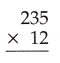2,820,

Explanation:
1
235
X 12
0470
2350
2,820

Question 2.3,652,

Explanation:
332
X 11
0332
3320
3,652

Question 3.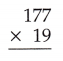3,363,

Explanation:
6,6
177
X 19
1,593
1,770
3,363

Question 4.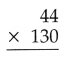5,720,

Explanation:
1
44
X 130
0000
1320
4400
5,720

Question 5.84,210,

Explanation:
401
X 210
00000
04010
80200
84,210

Question 6.31,049

Explanation:
5
509
X 61
00509
30540
31,049

Question 7.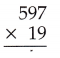11,343,

Explanation:
597
X 19
05373
05970
11,343

Question 8.17,922,

Explanation:
618
X 29
05,562
12,360
17,922

Question 9.
Ariel earns money by mowing lawns in his neighborhood. If he can mow 3 lawns in an hour, how many lawns can he mow working 30 hours a week?
90 lawns in a week,

Explanation:
Given Ariel earns money by mowing lawns in his neighborhood.
If he can mow 3 lawns in an hour, Number how many lawns can he mow working 30 hours a week is 30 X 3 lawns = 90 lawns in a week.

Question 10.
Winona is tracking the amount of water that the people of her town use during the summer months. She calculates that 47,005 gallons of water are used every day. If Wmona tracks the water usage for 112 days, how much water will be used during that time?
52,64,560 gallons of water,

Explanation:
As Winona is tracking the amount of water that the people of her town use during the summer months. She calculates that 47,005 gallons of water are used every day. If Wmona tracks the water usage for 112 days, how much water will be used during that time is 47,005 X 112 = 52,64,560 gallons of water.

Exercises Divide

Question 1.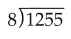156 R7,

Explanation:
Given to divide 1255 by 8 we get
8)1255(156
08
45
40
55
48
7
so it is 156R7.

Question 2.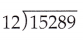1,274R1,

Explanation:
Given to divide 15,289 by 12 we get
12) 15,289(1,274
12
32
24
088
84
49
48
1
So it is 1,274R1.

Question 3.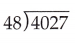83R43,

Explanation:
Given to divide 4,027 by 48 we get
48) 4027(83
384
187
144
43
So it is 83R43.

Question 4.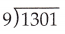144R5,

Explanation:
Given to divide 1,301 by 9 we get
9)1,301(144
09
40
36
41
36
So it is 144R5.

Question 5.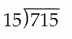47R10,

Explanation:
Given to divide 715 by 15 we get
15)715(47
60
115
105
10
So it is 47R10.

Question 6.33R7,

Explanation:
Given to divide 403 by 12 we get
12) 403(33
36
43
36
7
So it is 33R7.

Question 7.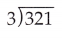107,

Explanation:
Given to divide 321 by 3 we get
3)321(107
30
21
21
0
So it is 107.

Question 8.85,

Explanation:
Given to divide 425 by 5 we get
5)425(85
40
25
25
0
So it is 85.

Question 9.
Aubrey wants to divide his penny collection equally among his 14 cousins. ¡f he has 1,722 pennies in his collection, how many pennies will each one of his cousinš receive?
Each one of his cousinš receive 123 pennies,

Explanation:
As Aubrey wants to divide his penny collection equally among his 14 cousins.
If he has 1,722 pennies in his collection, Number of pennies will each one of
his cousinš receive are 14 divides 1,722 are
14)1,722(123
14
32
28
42
42
0
123 pennies.

Question 10.
Emily wants to bring candy to share with her class at school. If she has 210 pieces of candy, and there are 30 students in her class, how many pieces of candy will each student receive?